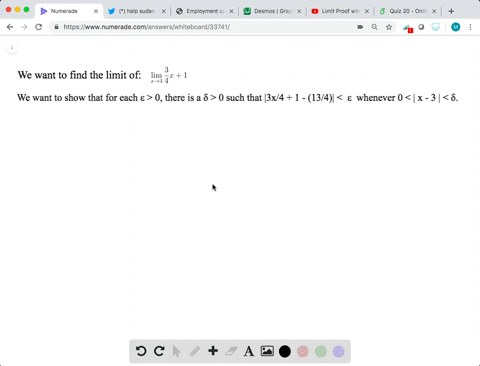Enroll in one of our FREE online STEM bootcamps. Join today and start acing your classes!View Bootcamps### Using the $\varepsilon$ -\delta Definition of Lim…

03:42University of California, Berkeley
Problem 47

# Using the $\varepsilon$ -\delta Definition of Limit In Exercises $45-56$ , find the limit $L$ . Then use the $\varepsilon-\delta$ definition to prove that the limit is $L$ .$$\lim _{x \rightarrow-4}\left(\frac{1}{2} x-1\right)$$

## Discussion

You must be signed in to discuss.

## Video Transcript

Okay, so we have the limits as X approaches make it four of 1/2. Excellent. One. If we were talking before we get there, then we can right that for every f longer than zero, there exists. Hey, felt a great is that our function minus l give us an excellent whenever euro is us then X minus R plus four, then don't. So let's see. So our function is 1/2 minus one, minus our limits. That was like the basic. That's plus three. This is equal to 1/2 ex put, too. You can factor out a one house. We get 1/2 after a lot of experts. What you said that experts for has to be left in Delta. But if it lefton 1/2 delta which is equal to Absalon. So solving for Epsilon we have that are solving for Delta, we have that Delta is equal to excellent. I'm still, uh, we need you have They're all less than a foot for But then delta, which is equal to two